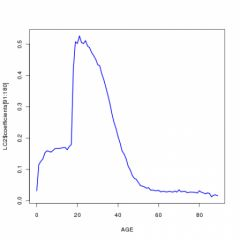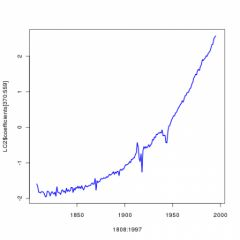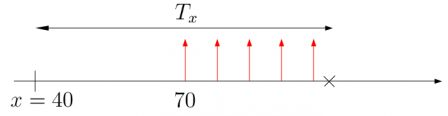Want to share your content on R-bloggers? click here if you have a blog, or here if you don't.The final course (since courses end this week in Montréal) can be watched here and there. The drawings from the course can be downloaded here (including last week’s). First, to come back on last week’s course , we considered
Lee-carter model, i.e.Note that it is possible to go a bit further, and to consider something that can be seen as a second order developmentor even more generallyThis idea was implicitly in the initial paper published in the 80’s. Hence, I mentioned in the course that (weighted) least square techniques can be considered to estimate parameters,and the the first order condition is simply(which was the interpretation that it is simply the average (over years) of mortality rate). Given that estimator, it is possible to writeand then to use the first components of the singular value decomposition to derive estimators.About the singular value decomposition (SVD, with a nice graph stolen from wikipedia, here, on the right – actually the French article on SVD is a bit more punchy, from Снайперская винтовка
Драгунова
, there). Note that SVD is closely related to PCA…
Thus, instead of focusing only on the first principal components, it is possible to go up to order 2.
But it is also possible to add, instead of a time component, a cohort-based component, i.e.The estimation is rather simple, as shown below

```D=as.vector(BASEB)
E=as.vector(BASEC)
A=rep(AGE,each=length(ANNEE))
Y=rep(ANNEE,length(AGE))
C=Y-A
base=data.frame(D,E,A,Y,C,a=as.factor(A),
y=as.factor(Y),c=as.factor(C))
LC4=gnm(D~a+Mult(a,y)+Mult(a,c),offset=log(E),
family=poisson,data=base)```

Here, parameters have the following shape.

```plot(AGE[-1],LC2\$coefficients[2:90])
plot(AGE,LC2\$coefficients[91:180])
plot(ANNEE,LC2\$coefficients[181:279])
plot(AGE,LC2\$coefficients[280:369])
plot(1808:1997,LC2\$coefficients[370:559])```

The first componentis rather similar to what we had beforeOn the other hand, the Lee-Carter (age-year) component isand the cohort effect (age-cohort) isNote that the year-component captures wars and chocks that can affect anyone some given years, and that gains on life expectancy is more a cohort effect.
Finally, we discussed actuarial applications. But first, recall that in actuarial literature (without any dynamics), it is standard to definedandThe mortality rate is theni.e.Thus,Complete expectation of life is thenor its discrete versionHence, it is possible to writewhich can be extended in the dynamic framework as followsA natural estimator of that quantity isi.e., with Lee-Carter modelAll those quantities can be computed quite simply.

But first, I have to work a little bit on the dataset, at least to be able to predict mortality up to 100 years old (with the demography package, we have to stop at 90). One idea can be to mix two estimation techniques: the nonlinear Poisson regression to getandup to 99 years old, and then to use Rob Hyndman’s package to estimate and predict thecomponent. But first, we have to check that‘s and‘s
with the two techniques are not too different. The code for the first model is

```BASEB=BASEB[,1:99]
BASEC=BASEC[,1:99]
AGE=AGE[1:99]
library(gnm)
D=as.vector(BASEB)
E=as.vector(BASEC)
A=rep(AGE,each=length(ANNEE))
Y=rep(ANNEE,length(AGE))
base=data.frame(D,E,A,Y,a=as.factor(A),
y=as.factor(Y))
LC2=gnm(D~a+Mult(a,y)+offset=log(E),
family=poisson,data=base)```

while it is

```BASEB=BASEB[,1:90]
BASEC=BASEC[,1:90]
AGE=AGE[1:90]
library(demography)
MU=as.matrix(BASEB/BASEC)
base=demogdata(data=t(MU),pop=t(BASEC),
ages=AGE,years=ANNEE,type="mortality",
label="France",name="Total",lambda=0)
LC3=lca(base,interpolate=TRUE)
LC3F=forecast(LC3,150)```

for the second one. Then, we can compare predictions for‘s e.g. (with output from econometric regression in blue, and Rob’s package in red)

```plot(AGE,LC3\$ax,lwd=2,col="red",xlab="",ylab="")
lines(1:98,LC2\$coefficients+LC2\$coefficients[2:99],
lwd=2,col="blue")```The second problem is that we have to use a linear transformation, since in the econometric package, we do not use the same constraints as in Rob’s package. Here, it was

```plot(ANNEE,-LC2\$coefficients[199:297]*50)
lines(ANNEE,LC3\$kt,col="red")```Then we can compute predictedfor all ages and years,

```A=LC2\$coefficients+LC2\$coefficients[2:99]
B=LC2\$coefficients[100:190]
K1=LC3\$kt
K2=LC3\$kt+LC3F\$kt.f\$mean
K=-c(K1,K2)/50
MU=matrix(NA,length(A),length(K))
for(i in 1:length(A)){
for(j in 1:length(K)){
MU[i,j]=exp(A[i]+B[i]*K[j])
}}```

Once we have that matrix, we simply have to work on diagonal (i.e. cohorts) to calculate anything. For instance, to get the dynamic version of, the code is (here for someone of age 40 in 2000)

```t=2000
x=40
s=seq(0,99-x-1)
MUd=MU[x+1+s,t+s-1898]
Pxt=cumprod(exp(-diag(MUd)))```

It is also possible to compute residual life expectancy, including dynamics

```x=40
E=rep(NA,150)
for(t in 1900:2040){
s=seq(0,90-x-1)
MUd=MU[x+1+s,t+s-1898]
Pxt=cumprod(exp(-diag(MUd)))
ext=sum(Pxt)
E[t-1899]=ext}
plot(1900:2049,E)```Note that this has been computed given the maximum lifetime we had in the dataset (here 99 years old). Hence, we assume here that no one can live more than 100 year (which will impact life expectancy and is a rather strong assumption, especially in 2050 – which might explain the concave shape of the curve on the right part).
It is also possible to compute annuities. For instance, consider a 40 years old insured, in 2000, willing to get 1 every year after age 70, until he dies, i.e.The annuity for such a contract is

```x=40
t=2000
r=.035
m=70
h=seq(0,21)
V=1/(1+r)^(m-x+h)*Pxt[m-x+h]
sum(V)
VV=rep(NA,150)

for(t in 1900:2040){
s=seq(0,90-x-1)
MUd=MU[x+1+s,t+s-1898]
Pxt=cumprod(exp(-diag(MUd)))
h=seq(0,30)
V=1/(1+r)^(m-x+h)*Pxt[m-x+h]
VV[t-1899]=sum(V,na.rm=TRUE)}
plot(1900:2049,VV)```(in order to compare the value of such a contract, from 1900 up to 2050).